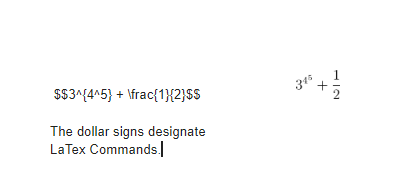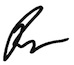This add-on lets you instantly convert every math equation in your document into beautiful latex images!

This add-on lets you automatically convert every LaTeX equation in your document into beautiful images! Simply enclose your math equations within $$…$$ and click the button in the sidebar, and all of your equations will be rendered in LaTeX!

LaTex is way to write equations as plain text, which are then converted to a graphical representation of the equation. For example:In this example 3^{4^5} + \frac{1}{2} is the LaTeX command, and is wrapped in double dollar signs so the add-on can render the equation.

There are tons of resources on learning how to write LaTeX, and if you have any students going into scientific or mathematics careers, learning LaTeX now will give them a step up on their peers.Be sure to subscribe to my YouTube channel and join your fellow educators on the Eduk8me email list!